Fitting Curves

# Parametric Regression Fits

Fitting a curve produces a visual display that reflects the systematic variation of the data. In this section, you will fit polynomial curves using a subset of the MINING data set described in Chapter 1, "Getting Started."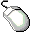Open the MININGX data set.Choose Analyze:Fit ( Y X ).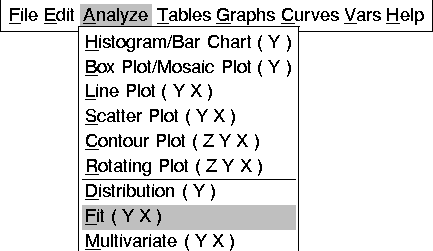The fit variables dialog appears, as shown in Figure 13.3.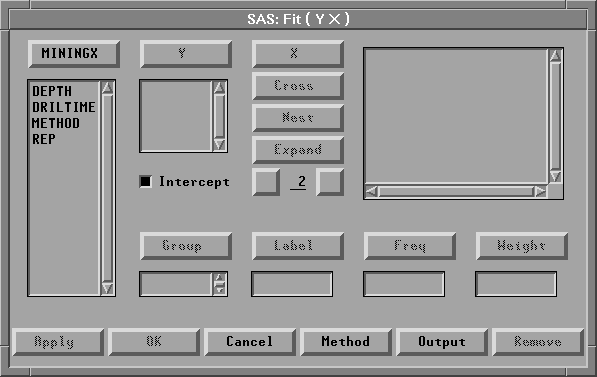Figure 13.3: Fit Variables DialogSelect the variable DRILTIME, then click the Y button.

DRILTIME appears in the Y variables list.Select the variable DEPTH, then click the X button.

DEPTH appears in the X variables list.Click the Output button.

The fit output options dialog, shown in Figure 13.4, appears on your display.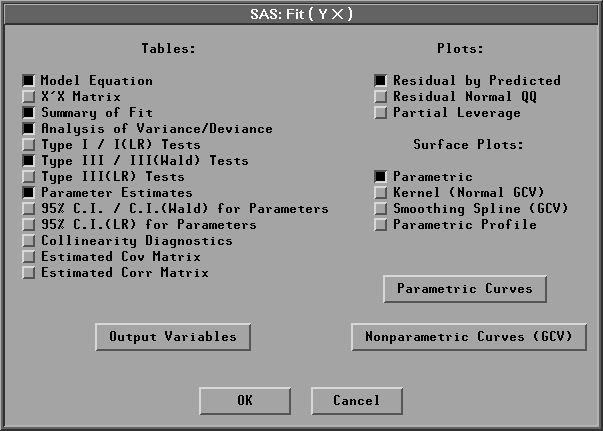Figure 13.4: Fit Output Options Dialog

In the output options dialog, you specify which curves and tables will appear in the fit window. The default curve is a polynomial of degree one, that is, a line. The options set by default in this dialog are appropriate aids to a careful modeling of the data. They are not needed here where the purpose is to produce a visual display that reflects the trend of the data.Turn off all check boxes by clicking on any that are highlighted.Click the OK button in all dialogs.

A fit window appears, as shown in Figure 13.5.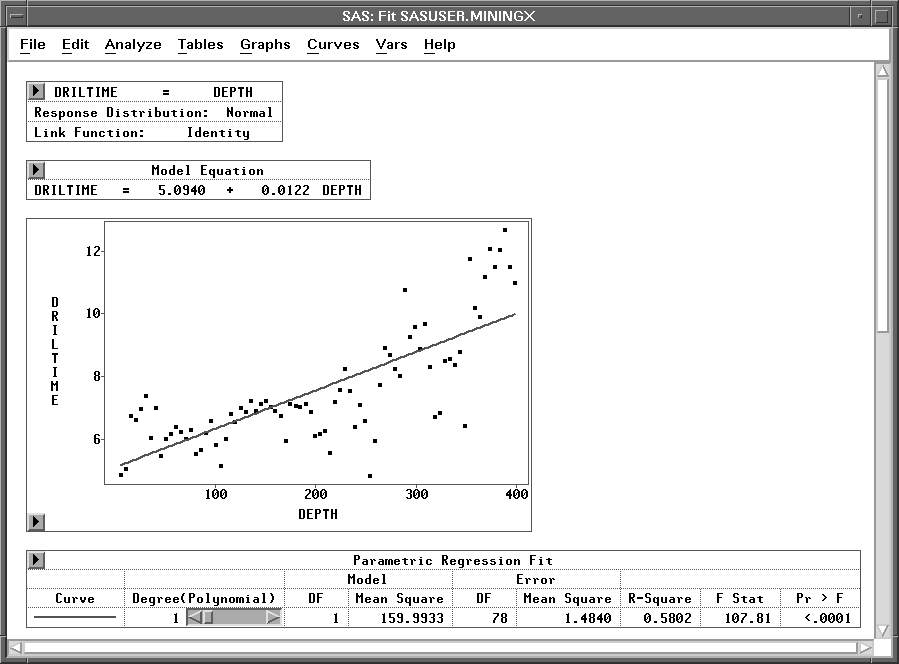Figure 13.5: Fit Window with Line

The fit window contains a plot of DRILTIME by DEPTH along with a table summarizing the fit. A simple regression line is superimposed on the plot; it follows the linear trend of the data. Notice, though, that the plot shows curvature that a straight line cannot follow.

First examine the Parametric Regression Fit table corresponding to these data. The R-Square value is 0.5802, which means that 58% of the variation in drilling times is explained by DEPTH. The rest of this table contains statistics pertinent to hypothesis testing, and they are discussed in Chapter 14, "Multiple Regression."